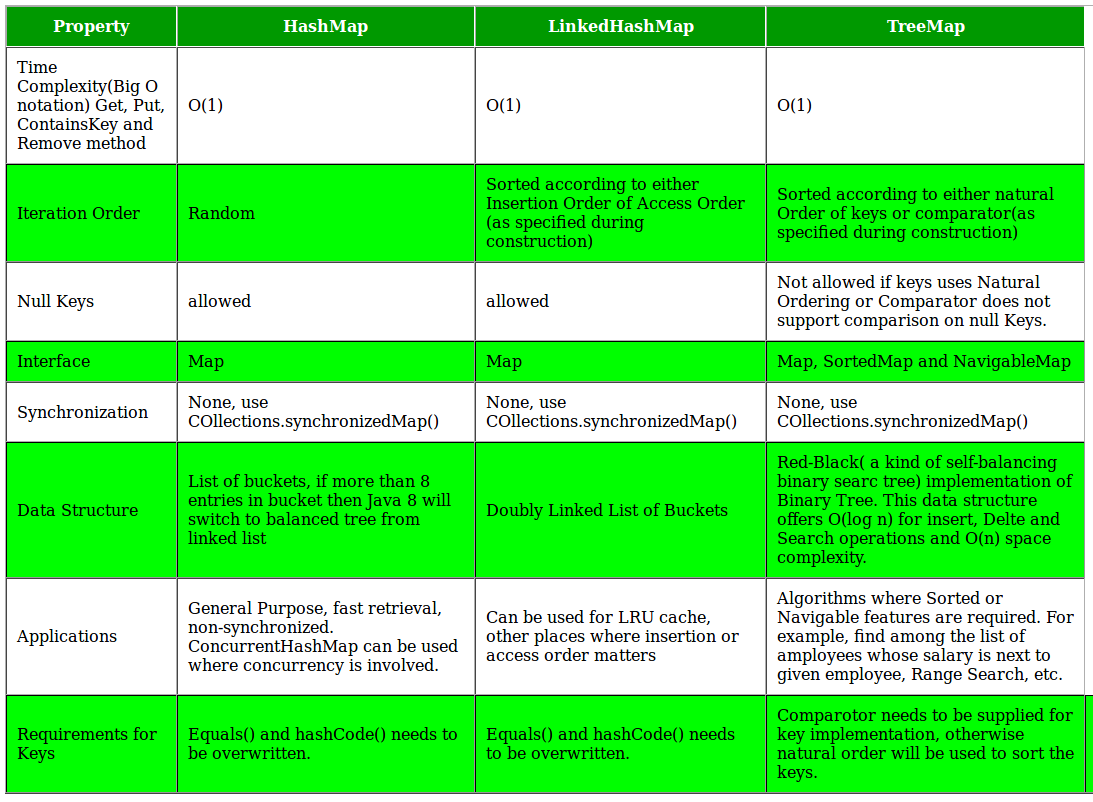Related Articles
Differences between TreeMap, HashMap and LinkedHashMap in Java
• Difficulty Level : Medium
• Last Updated : 11 Dec, 2018

Prerequisite : HashMap and TreeMap in Java

TreeMap, HashMap and LinkedHashMap: What’s Similar?

• All offer a key->value map and a way to iterate through the keys. The most important distinction between these classes is the time guarantees and the ordering of the keys.
• All three classes HashMap, TreeMap and LinkedHashMap implements java.util.Map interface, and represents mapping from unique key to values.

Key Points

1. HashMap: HashMap offers 0(1) lookup and insertion. If you iterate through the keys, though, the ordering of the keys is essentially arbitrary. It is implemented by an array of linked lists.
Syntax:

```public class HashMap extends AbstractMap
implements Map,Cloneable, Serializable```
• A HashMap contains values based on the key.
• It contains only unique elements.
• It may have one null key and multiple null values.
• It maintains no order.
2. LinkedHashMap: LinkedHashMap offers 0(1) lookup and insertion. Keys are ordered by their insertion order. It is implemented by doubly-linked buckets.
Syntax:

```public class LinkedHashMap extends HashMap
0implements Map```
• A LinkedHashMap contains values based on the key.
• It contains only unique elements.
• It may have one null key and multiple null values.
• It is same as HashMap instead maintains insertion order.
3. TreeMap: TreeMap offers O(log N) lookup and insertion. Keys are ordered, so if you need to iterate through the keys in sorted order, you can. This means that keys must implement the Comparable interface. TreeMap is implemented by a Red-Black Tree.
Syntax:

```public class TreeMap extends AbstractMap implements
NavigableMap, Cloneable, Serializable```
• A TreeMap contains values based on the key. It implements the NavigableMap interface and extends AbstractMap class.
• It contains only unique elements.
• It cannot have null key but can have multiple null values.
• It is same as HashMap instead maintains ascending order(Sorted using the natural order of its key).
4. Hashtable: “Hashtable” is the generic name for hash-based maps.
Syntax:

```public class Hashtable extends Dictionary implements
Map, Cloneable, Serializable```
• A Hashtable is an array of list. Each list is known as a bucket. The position of bucket is identified by calling the hashcode() method. A Hashtable contains values based on the key.
• It contains only unique elements.
• It may have not have any null key or value.
• It is synchronized.
• It is a legacy class.

## HashMap

 `// Java program to print ordering  ` `// of all elements using HashMap ` `import` `java.util.*; ` `import` `java.lang.*; ` `import` `java.io.*; ` `class` `Main ` `{ ` `    ``// This function prints ordering of all elements ` `    ``static` `void` `insertAndPrint(AbstractMap map) ` `    ``{  ` `        ``int``[] array= {``1``, -``1``, ``0``, ``2``,-``2``}; ` `        ``for` `(``int` `x: array)  ` `        ``{  ` `            ``map.put(x, Integer.toString(x));  ` `        ``}  ` `        ``for` `(``int` `k: map.keySet()) ` `        ``{ ` `            ``System.out.print(k + ``", "``);  ` `        ``} ` `    ``}  ` ` `  `    ``// Driver method to test above method ` `    ``public` `static` `void` `main (String[] args) ` `    ``{ ` `        ``HashMap map = ``new` `HashMap(); ` `        ``insertAndPrint(map); ` `    ``} ` `} `

 `// Java program to print ordering  ` `// of all elements using LinkedHashMap ` `import` `java.util.*; ` `import` `java.lang.*; ` `import` `java.io.*; ` ` `  `class` `Main ` `{ ` `    ``// This function prints ordering of all elements ` `    ``static` `void` `insertAndPrint(AbstractMap map) ` `    ``{  ` `        ``int``[] array= {``1``, -``1``, ``0``, ``2``,-``2``}; ` `        ``for` `(``int` `x: array)  ` `        ``{  ` `            ``map.put(x, Integer.toString(x));  ` `        ``}  ` `        ``for` `(``int` `k: map.keySet()) ` `        ``{ ` `            ``System.out.print(k + ``", "``);  ` `        ``} ` `    ``}  ` `     `  `    ``// Driver method to test above method ` `    ``public` `static` `void` `main (String[] args) ` `    ``{ ` `        ``LinkedHashMap map = ``new` `LinkedHashMap(); ` `        ``insertAndPrint(map); ` `    ``} ` `} `

## TreeMap

 `// Java program to print ordering of ` `// all elements using TreeMap ` ` `  `import` `java.util.*; ` `import` `java.lang.*; ` `import` `java.io.*; ` ` `  `class` `Main ` `{ ` `    ``// This function prints ordering of all elements ` `    ``static` `void` `insertAndPrint(AbstractMap map) ` `    ``{  ` `        ``int``[] array= {``1``, -``1``, ``0``, ``2``,-``2``}; ` `        ``for` `(``int` `x: array)  ` `        ``{  ` `            ``map.put(x, Integer.toString(x));  ` `        ``}  ` `        ``for` `(``int` `k: map.keySet()) ` `        ``{ ` `            ``System.out.print(k + ``", "``);  ` `        ``} ` `    ``}  ` ` `  `    ``// Driver method to test above method ` `    ``public` `static` `void` `main (String[] args) ` `    ``{ ` `        ``TreeMap map = ``new` `TreeMap(); ` `        ``insertAndPrint(map); ` `    ``} ` `} `

Output of HashMap:

```-1, 0, 1, -2, 2,
// ordering of the keys is essentially arbitrary (any ordering)
```

``` 1, -1, 0, 2, -2,
// Keys are ordered by their insertion order
```

Output of TreeMap:

``` -2, -1, 0, 1, 2,
// Keys are in sorted order
```

Comparison TableReal Life Applications

1. Suppose you were creating a mapping of names to Person objects. You might want to periodically output the people in alphabetical order by name. A TreeMap lets you do this.
2. A TreeMap also offers a way to, given a name, output the next 10 people. This could be useful for a “More”function in many applications.
3. A LinkedHashMap is useful whenever you need the ordering of keys to match the ordering of insertion. This might be useful in a caching situation, when you want to delete the oldest item.
4. Generally, unless there is a reason not to, you would use HashMap. That is, if you need to get the keys back in insertion order, then use LinkedHashMap. If you need to get the keys back in their true/natural order, then use TreeMap. Otherwise, HashMap is probably best. It is typically faster and requires less overhead.

This article is contributed by Mr. Somesh Awasthi. If you like GeeksforGeeks and would like to contribute, you can also write an article using contribute.geeksforgeeks.org or mail your article to contribute@geeksforgeeks.org. See your article appearing on the GeeksforGeeks main page and help other Geeks.